GFG App
Open AppBrowser
Continue

# Basic Operators in Shell Scripting

There are 5 basic operators in bash/shell scripting:

• Arithmetic Operators
• Relational Operators
• Boolean Operators
• Bitwise Operators
• File Test Operators

1. Arithmetic Operators: These operators are used to perform normal arithmetics/mathematical operations. There are 7 arithmetic operators:

• Subtraction (-): Binary operation used to subtract two operands.
• Multiplication (*): Binary operation used to multiply two operands.
• Division (/): Binary operation used to divide two operands.
• Modulus (%): Binary operation used to find remainder of two operands.
• Increment Operator (++): Unary operator used to increase the value of operand by one.
• Decrement Operator (- -): Unary operator used to decrease the value of a operand by one

## C

 `#!/bin/bash ` ` `  `#reading data from the user ` `read - p ``'Enter a : '` `a ` `           ``read ` `    ``- p ``'Enter b : '` `b ` ` `  `          ``add ` `    ``= \$((a + b)) ` `        ``echo Addition of a and b are \$add ` ` `  `            ``sub ` `    ``= \$((a - b)) ` `        ``echo Subtraction of a and b are \$sub ` ` `  `            ``mul ` `    ``= \$((a * b)) ` `        ``echo Multiplication of a and b are \$mul ` ` `  `            ``div` `    ``= \$((a / b)) ` `        ``echo division of a and b are \$``div` ` `  `            ``mod ` `    ``= \$((a % b)) ` `          ``echo Modulus of a ` `      ``and b are \$mod ` ` `  `      ``((++a)) ` `          ``echo Increment ` `          ``operator when applied on ``"a"` `results into a = \$a ` ` `  `      ``((--b)) ` `          ``echo Decrement ` `          ``operator when applied on ``"b"` `results into b = \$b `

Output: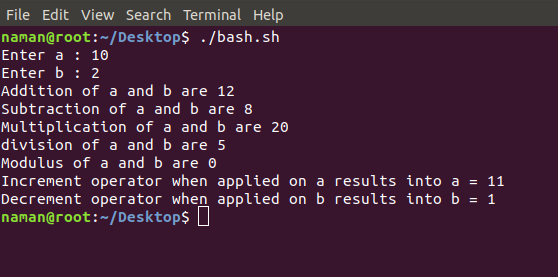2. Relational Operators: Relational operators are those operators which define the relation between two operands. They give either true or false depending upon the relation. They are of 6 types:

• ‘==’ Operator: Double equal to operator compares the two operands. Its returns true is they are equal otherwise returns false.
• ‘!=’ Operator: Not Equal to operator return true if the two operands are not equal otherwise it returns false.
• ‘<‘ Operator: Less than operator returns true if first operand is less than second operand otherwise returns false.
• ‘<=’ Operator: Less than or equal to operator returns true if first operand is less than or equal to second operand otherwise returns false
• ‘>’ Operator: Greater than operator return true if the first operand is greater than the second operand otherwise return false.
• ‘>=’ Operator: Greater than or equal to operator returns true if first operand is greater than or equal to second operand otherwise returns false

## C

 `#!/bin/bash ` `  `  `#reading data from the user ` `read -p ``'Enter a : '` `a ` `read -p ``'Enter b : '` `b ` `  `  `if``(( \$a==\$b )) ` `then ` `    ``echo a is equal to b. ` `else` `    ``echo a is not equal to b. ` `fi ` `  `  `if``(( \$a!=\$b )) ` `then ` `    ``echo a is not equal to b. ` `else` `    ``echo a is equal to b. ` `fi ` `  `  `if``(( \$a<\$b )) ` `then ` `    ``echo a is less than b. ` `else` `    ``echo a is not less than b. ` `fi ` `  `  `if``(( \$a<=\$b )) ` `then ` `    ``echo a is less than or equal to b. ` `else` `    ``echo a is not less than or equal to b. ` `fi ` `  `  `if``(( \$a>\$b )) ` `then ` `    ``echo a is greater than b. ` `else` `    ``echo a is not greater than b. ` `fi ` `  `  `if``(( \$a>=\$b )) ` `then ` `    ``echo a is greater than or equal to b. ` `else` `    ``echo a is not greater than or equal to b. ` `fi `

Output: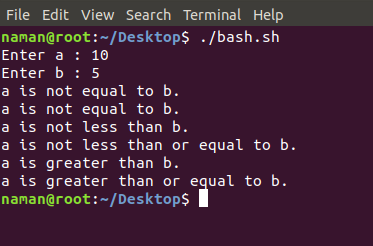3. Logical Operators : They are also known as boolean operators. These are used to perform logical operations. They are of 3 types:

• Logical AND (&&): This is a binary operator, which returns true if both the operands are true otherwise returns false.
• Logical OR (||): This is a binary operator, which returns true is either of the operand is true or both the operands are true and return false if none of then is false.
• Not Equal to (!): This is a unary operator which returns true if the operand is false and returns false if the operand is true.

## C

 `#!/bin/bash ` ` `  `#reading data from the user ` `read -p ``'Enter a : '` `a ` `read -p ``'Enter b : '` `b ` ` `  `if``((\$a == ``"true"` `& \$b == ``"true"` `)) ` `then ` `    ``echo Both are ``true``. ` `else` `    ``echo Both are not ``true``. ` `fi ` ` `  `if``((\$a == ``"true"` `|| \$b == ``"true"` `)) ` `then ` `    ``echo Atleast one of them is ``true``. ` `else` `    ``echo None of them is ``true``. ` `fi ` ` `  `if``(( ! \$a == ``"true"`  `)) ` `then ` `    ``echo ``"a"` `was initially ``false``. ` `else` `     ``echo ``"a"` `was initially ``true``. ` ` ``fi `

Output: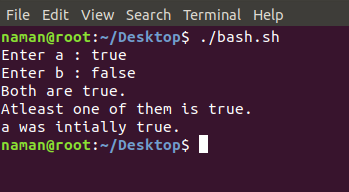4. Bitwise Operators: A bitwise operator is an operator used to perform bitwise operations on bit patterns. They are of 6 types:

• Bitwise And (&): Bitwise & operator performs binary AND operation bit by bit on the operands.
• Bitwise OR (|): Bitwise | operator performs binary OR operation bit by bit on the operands.
• Bitwise XOR (^): Bitwise ^ operator performs binary XOR operation bit by bit on the operands.
• Bitwise complement (~): Bitwise ~ operator performs binary NOT operation bit by bit on the operand.
• Left Shift (<<): This operator shifts the bits of the left operand to left by number of times specified by right operand.
• Right Shift (>>): This operator shifts the bits of the left operand to right by number of times specified by right operand.

## C

 `#!/bin/bash ` ` `  `#reading data from the user ` `read -p ``'Enter a : '` `a ` `read -p ``'Enter b : '` `b ` ` `  `bitwiseAND=\$(( a&b )) ` `echo Bitwise AND of a and b is \$bitwiseAND ` ` `  `bitwiseOR=\$(( a|b )) ` `echo Bitwise OR of a and b is \$bitwiseOR ` ` `  `bitwiseXOR=\$(( a^b )) ` `echo Bitwise XOR of a and b is \$bitwiseXOR ` ` `  `bitiwiseComplement=\$(( ~a )) ` `echo Bitwise Compliment of a is \$bitiwiseComplement ` ` `  `leftshift=\$(( a<<1 )) ` `echo Left Shift of a is \$leftshift ` ` `  `rightshift=\$(( b>>1 )) ` `echo Right Shift of b is \$rightshift `

Output: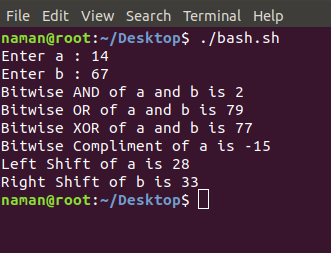5. File Test Operator: These operators are used to test a particular property of a file.

• -b operator: This operator check whether a file is a block special file or not. It returns true if the file is a block special file otherwise false.
• -c operator: This operator checks whether a file is a character special file or not. It returns true if it is a character special file otherwise false.
• -d operator: This operator checks if the given directory exists or not. If it exists then operators returns true otherwise false.
• -e operator: This operator checks whether the given file exists or not. If it exits this operator returns true otherwise false.
• -r operator: This operator checks whether the given file has read access or not. If it has read access then it returns true otherwise false.
• -w operator: This operator check whether the given file has write access or not. If it has write then it returns true otherwise false.
• -x operator: This operator check whether the given file has execute access or not. If it has execute access then it returns true otherwise false.
• -s operator: This operator checks the size of the given file. If the size of given file is greater than 0 then it returns true otherwise it is false.

## C

 `#!/bin/bash ` ` `  `#reading data from the user ` `read -p ``'Enter file name : '` `FileName ` ` `  `if` `[ -e \$FileName ] ` `then ` `    ``echo File Exist ` `else` `    ``echo File doesnot exist ` `fi ` ` `  `if` `[ -s \$FileName ] ` `then ` `    ``echo The given file is not empty. ` `else` `    ``echo The given file is empty. ` `fi ` ` `  `if` `[ -r \$FileName ] ` `then ` `    ``echo The given file has read access. ` `else` `    ``echo The given file does not has read access. ` `fi ` ` `  `if` `[ -w \$FileName ] ` `then ` `    ``echo The given file has write access. ` `else` `    ``echo The given file does not has write access. ` `fi ` ` `  `if` `[ -x \$FileName ] ` `then ` `    ``echo The given file has execute access. ` `else` `    ``echo The given file does not has execute access. ` `fi `

Output: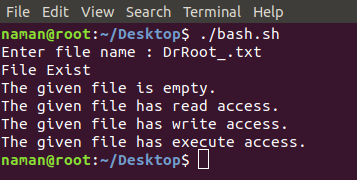My Personal Notes arrow_drop_up